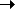Package net.flashpunk.tweens.motion Class public class CircularMotion Inheritance CircularMotionMotionTweenObject

Determines a circular motion.

Public Properties
PropertyDefined Byactive : Boolean
If the tween should update.
Tween
angle : Number
[read-only] The current position on the circle.
CircularMotion
circumference : Number
[read-only] The circumference of the current circle motion.
CircularMotioncomplete : Function
Tween completion callback.
Tweendelay : Number = 0
Length of time to wait before starting this tween.
Tweenobject : Object
Target object for the tween.
Motionpercent : Number
The completion percentage of the Tween.
Tweenscale : Number
[read-only] The current time scale of the Tween (after easer has been applied).
Tweenx : Number
Current x position of the Tween.
Motiony : Number
Current y position of the Tween.
Motion
Protected Properties
PropertyDefined By_object : Object
Motion_x : Number = 0
Motion_y : Number = 0
Motion
Public Methods
MethodDefined By

CircularMotion(complete:Function = null, type:uint = 0)
Constructor.
CircularMotioncancel():void
Immediately stops the Tween and removes it from its Tweener without calling the complete callback.
Tween

setMotion(centerX:Number, centerY:Number, radius:Number, angle:Number, clockwise:Boolean, duration:Number, ease:Function = null):void
Starts moving along a circle.
CircularMotion

setMotionSpeed(centerX:Number, centerY:Number, radius:Number, angle:Number, clockwise:Boolean, speed:Number, ease:Function = null):void
Starts moving along a circle at the speed.
CircularMotionstart():void
Starts the Tween, or restarts it if it's currently running.
Tweenupdate():void
Updates the Tween, called by World.
Tween
Public Constants
ConstantDefined ByLOOPING : uint = 1
[static] Looping Tween type, will restart immediately when it finishes.
TweenONESHOT : uint = 2
[static] Oneshot Tween type, will stop and remove itself from its core container when it finishes.
TweenPERSIST : uint = 0
[static] Persistent Tween type, will stop when it finishes.
Tween
Property Detail
 angle property
`angle:Number`  [read-only]

The current position on the circle.

Implementation
`    public function get angle():Number`
 circumference property
`circumference:Number`  [read-only]

The circumference of the current circle motion.

Implementation
`    public function get circumference():Number`
Constructor Detail
 CircularMotion () Constructor
`public function CircularMotion(complete:Function = null, type:uint = 0)`

Constructor.

Parameters
 `complete:Function` (default = `null`) — Optional completion callback. `type:uint` (default = `0`) — Tween type.
Method Detail
 setMotion () method
` public function setMotion(centerX:Number, centerY:Number, radius:Number, angle:Number, clockwise:Boolean, duration:Number, ease:Function = null):void`

Starts moving along a circle.

Parameters

 `centerX:Number` — X position of the circle's center. `centerY:Number` — Y position of the circle's center. `radius:Number` — Radius of the circle. `angle:Number` — Starting position on the circle. `clockwise:Boolean` — If the motion is clockwise. `duration:Number` — Duration of the movement. `ease:Function` (default = `null`) — Optional easer function.

 setMotionSpeed () method
` public function setMotionSpeed(centerX:Number, centerY:Number, radius:Number, angle:Number, clockwise:Boolean, speed:Number, ease:Function = null):void`

Starts moving along a circle at the speed.

Parameters

 `centerX:Number` — X position of the circle's center. `centerY:Number` — Y position of the circle's center. `radius:Number` — Radius of the circle. `angle:Number` — Starting position on the circle. `clockwise:Boolean` — If the motion is clockwise. `speed:Number` — Speed of the movement. `ease:Function` (default = `null`) — Optional easer function.